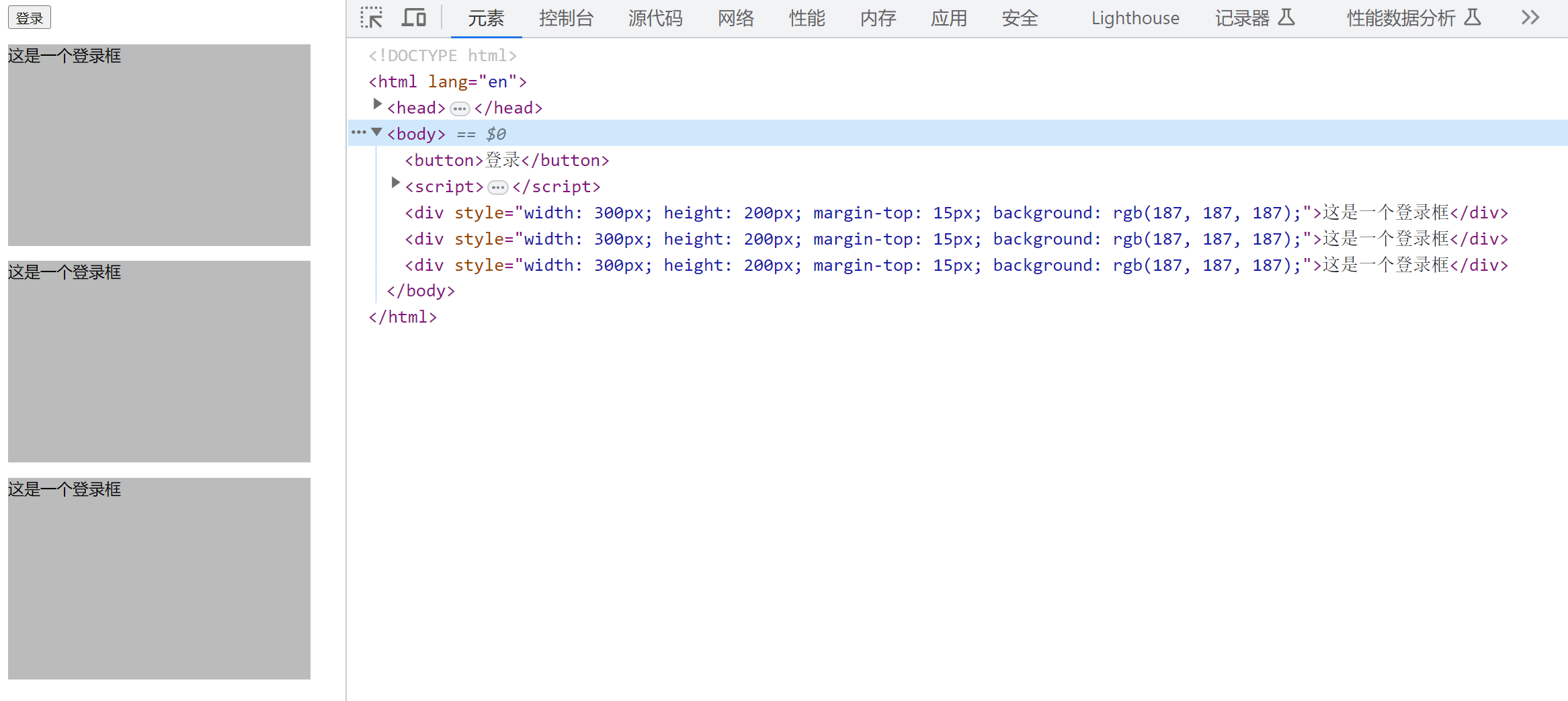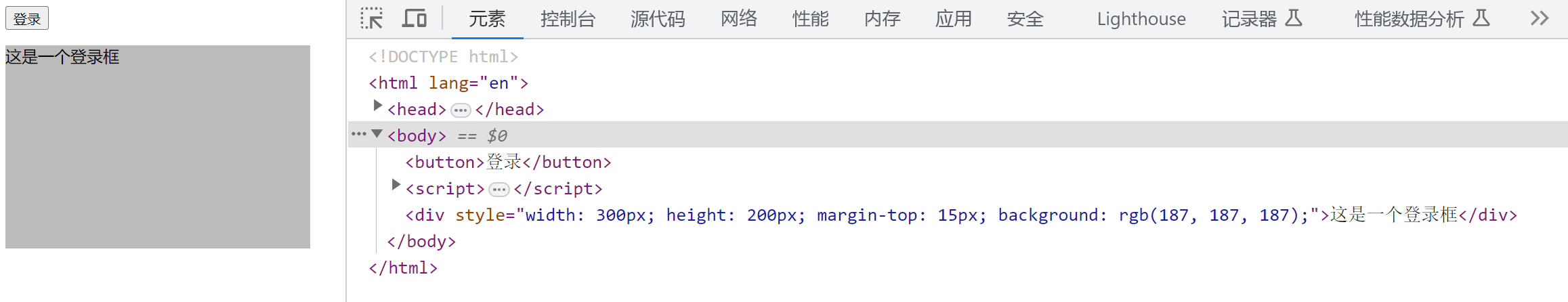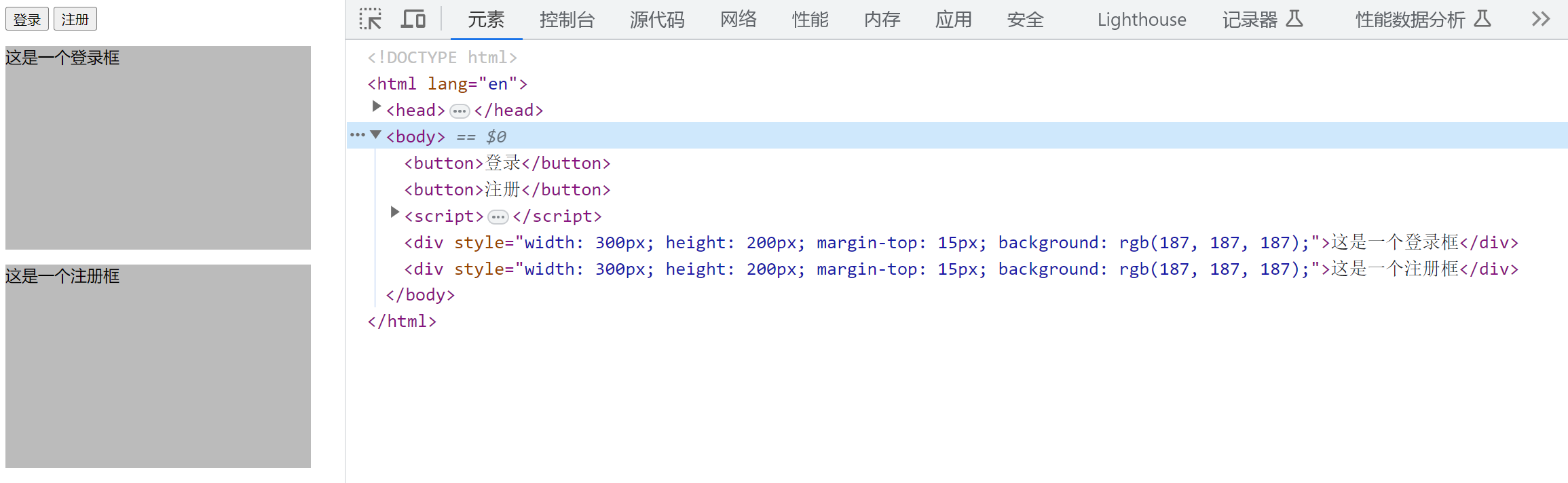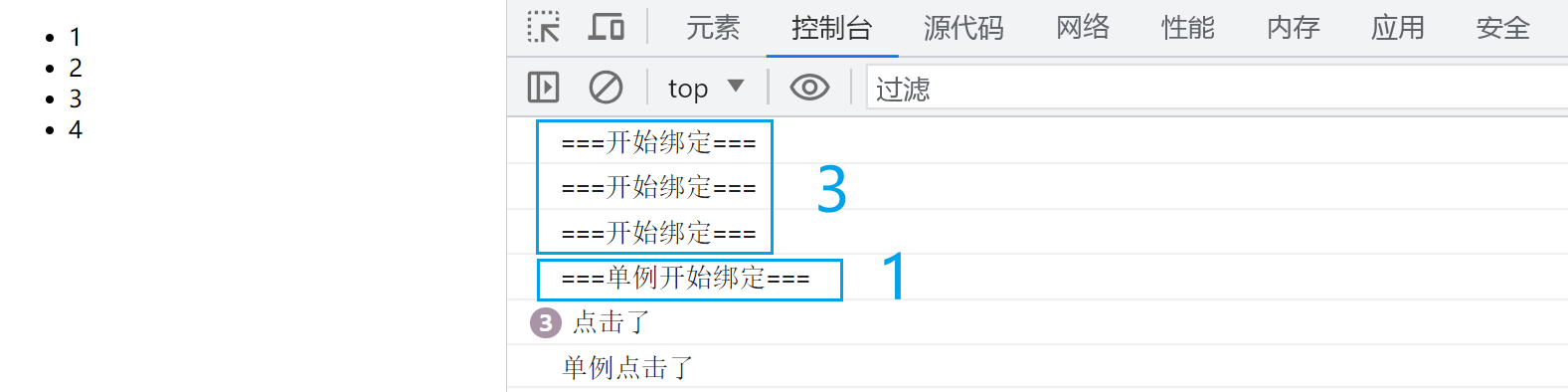# 设计模式之——单例模式

✍🏼作者：周棋洛，计算机学生
♉星座：金牛座
🏠主页：点击学习更多
🌐关键：JavaScript 单例 设计模式

#### 文章目录## 一、引言 🎉## 二、实现单例 ✌️

let Singleton = function (name) {
this.name = name;
}
Singleton.getInstance = (function () {
// 闭包，实例标记
let instance = null;
return function (name) {
if (!instance) {
instance = new Singleton(name);
}
return instance;
}
})();


// 测试
let a = Singleton.getInstance("王子周棋洛");
let b = Singleton.getInstance("王子");

console.log(a === b); // true


 let n1 =  new Singleton("周棋洛");
let n2 =  new Singleton("周棋洛王子");

console.log(n1 === n2); // false## 三、透明的单例 🪟

 let createP = (function () {
let instance;
// 重写构造函数
let createP = function (text) {
if (instance) {
return instance;
}
this.text = text;
this.init();
return instance = this;
}

createP.prototype.init = function () {
let p = document.createElement('p');
p.innerHTML = this.text;
document.body.appendChild(p);

}

// 返回新的构造函数
return createP;
})()

// 测试
let a = new createP('你好');
let b = new createP('世界');

console.log(a === b); // true


// 重写构造函数，闭包
let createP = function (text) {
if (instance) {
return instance;
}
this.text = text;
this.init();
return instance = this;
}


“单一职责原则”：简单来说，就是一个类或者模块应该只负责一项职责，不要承担过多的职责，以达到代码更加清晰、简洁、易于维护的目的。## 四、代理实现单例 ⛏️

 // 单一职责，负责创建dom
let createP = function (text) {
this.text = text;
this.init();
}

createP.prototype.init = function () {
let p = document.createElement('p');
p.innerHTML = this.text;
document.body.appendChild(p);
}

// 引入代理类，闭包，单实例
let ProxySingletonCreateP = (function () {
let instance;
return function (text) {
if (!instance) {
instance = new createP(text);
}
return instance;
}
})();

// 测试
let a = new ProxySingletonCreateP('你好');
let b = new ProxySingletonCreateP('世界');

console.log(a === b); // true## 五、JavaScript中的单例 📦

JavaScript 对象的创建底层包含了四个步骤：创建新的空对象、设置对象原型、将 proto 属性指向该原型对象、执行构造函数或者直接返回字面量对象。

let a = {}


let namespace1 = {
a:10,
b:20
};


 let user = (function () {
let _name = '王子';
return {
getUserInfo: function () {
return _name;
}
}
})();

console.log(user.getUserInfo()); // 王子## 六、惰性单例 🍕<body>
<button>登录</button>

<script>
let div = document.createElement("div");
div.style.width = "300px";
div.style.height = "200px";
div.style.marginTop = "15px";
div.style.background = "#bbb";
div.innerHTML = "这是一个登录框";
document.body.appendChild(div);
}

})
</script>
</body><body>
<button>登录</button>

<script>
let createLoginBox = function () {
let box;
return function () {
if (!box) {
let div = document.createElement("div");
div.style.width = "300px";
div.style.height = "200px";
div.style.marginTop = "15px";
div.style.background = "#bbb";
div.innerHTML = "这是一个登录框";
document.body.appendChild(div);
box = div;
}
return box;
}
}();

})
</script>
</body>## 七、通用惰性单例 🥛

 let getSingleton = function (fn) {
let instance;
return function () {
// 如果实例存在，直接返回，否则调用函数并给instanc赋值
return instance || (instance = fn.apply(this, arguments));
}
}


this 在 apply() 中指向的是当前上下文中的 this 对象，也就是 getSingleton 函数本身。

<body>
<button>登录</button>
<button>注册</button>

<script>
let createLoginBox = function () {
let div = document.createElement("div");
div.style.width = "300px";
div.style.height = "200px";
div.style.marginTop = "15px";
div.style.background = "#bbb";
div.innerHTML = "这是一个登录框";
document.body.appendChild(div);
return div;
};

let createRegisterBox = function () {
let div = document.createElement("div");
div.style.width = "300px";
div.style.height = "200px";
div.style.marginTop = "15px";
div.style.background = "#bbb";
div.innerHTML = "这是一个注册框";
document.body.appendChild(div);
return div;
};

let getSingleton = function (fn) {
let instance;
return function () {
// 如果实例存在，直接返回，否则调用函数并给instanc赋值
return instance || (instance = fn.apply(this, arguments));
}
}

let createSingletonRegisterBox = getSingleton(createRegisterBox);

})
createSingletonRegisterBox();
})
</script>
</body><body>
<ul>
<li>1</li>
<li>2</li>
<li>3</li>
<li>4</li>
</ul>

<script>
let getSingleton = function (fn) {
let instance;
return function () {
// 如果实例存在，直接返回，否则调用函数并给instanc赋值
return instance || (instance = fn.apply(this, arguments));
}
}

let bindEvent = function () {
console.log("===开始绑定===");
document.querySelectorAll("li").forEach(li => {
console.log("点击了")
})
})
return true;
}

let bindEventSingle = getSingleton(() => {
console.log("===单例开始绑定===");
document.querySelectorAll("li").forEach(li => {
console.log("单例点击了")
})
})
return true;
})

bindEvent();
bindEvent();
bindEvent();

bindEventSingle();
bindEventSingle();
bindEventSingle();
</script>
</body>## 八、总结 📄

03-20
08-271093
04-22406
05-1070
07-111844
04-1698万+
04-22662
05-113113
04-223258
03-16405
09-2050
04-131990
06-301409
09-16927
12-251988
08-18240

### “相关推荐”对你有帮助么？

•非常没帮助
•没帮助
•一般
•有帮助
•非常有帮助被折叠的  条评论 为什么被折叠?到【灌水乐园】发言¥1 ¥2 ¥4 ¥6 ¥10 ¥20获取中扫码支付点击重新获取扫码支付1.余额是钱包充值的虚拟货币，按照1:1的比例进行支付金额的抵扣。
2.余额无法直接购买下载，可以购买VIP、付费专栏及课程。余额充值